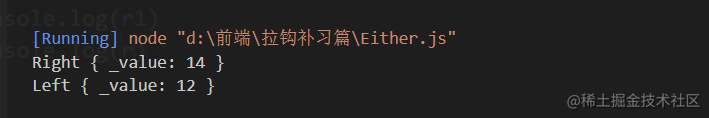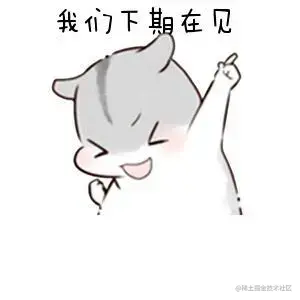# 【前端进阶】解决maybe函子问题的神奇函子 --- Either函子

## 什么是`Either`函子

• `Either`两者中的任何一个，类似于`if...else...`的处理
• 异常会让函数变得不纯，`Either`函子可以用来做异常处理

## 开始处理

### 1. 需求确定

`````````js
class left {
...
}
class right {
...
}
```

### 2. 类编写

`````````js
class Left {
static of (value) {
return new Left(value)
}
constructor (value) {
this._value = value
}
map (fn) {
return this
}
}
class Right {
static of (value) {
return new Right(value)
}
constructor (value) {
this._value = value
}
map (fn) {
return Right.of(fn(this._value))
}
}
```

### 3. 函子的区别

`````````js
let r1 = Right.of(12).map(x => x + 2)
let r2 = Left.of(12).map(x => x + 2)
console.log(r1)
console.log(r2)
```我们可以看到`Right`中的值是 `12+2 = 14`正确的输出的，但是`Left`却是返回了原来的参数，主要是因为`Left`中的`map`是直接返回了`this`

``````Left直接返回原来的函子，用来捕捉异常，Right用来在没有异常的时候执行输入的fn并返回一个函子。

**为什么要这么做呢？**

### 4. 为什么需要两种函子

``````为了解决上面的疑问，来个例子给大家演示一下，方便加深理解

```js
function parseJSON (str) {
try {
return Right.of(JSON.parse(str))
} catch (e) {
return Left.of({ error: e.message})
}
}
// 会出现错误的情况
let r = parseJSON('{ name: zs}')
console.log(r)
let r1 = parseJSON(JSON.stringify({ name:'zs'} ))
console.log(r)
```
![image.png](https://p1-juejin.byteimg.com/tos-cn-i-k3u1fbpfcp/472abe33d8004bb9998ddfd576a1fc5f~tplv-k3u1fbpfcp-watermark.image?)

## 完整代码

``````class Left {
static of (value) {
return new Left(value)
}
constructor (value) {
this._value = value
}
map (fn) {
return this
}
}
class Right {
static of (value) {
return new Right(value)
}
constructor (value) {
this._value = value
}
map (fn) {
return Right.of(fn(this._value))
}
}
//定义一个函数
function parseJSON (str) {
try {
return Right.of(JSON.parse(str))
} catch (e) {
//用来存储错误信息
return Left.of({ error: e.message})
}
}
// 传入错误的数据
let r = parseJSON('{ name: zs}')
console.log(r)
// 传入正确的数据
let r1 = parseJSON(JSON.stringify({ name:'zs'} ))
console.log(r1)
let r3 = Right.of(12).map(x => x + 2)
console.log(r3)
let r4 = Left.of(12).map(x => x + 2)
console.log(r4)

## 总结